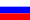# Vestnik KRAUNC. Fiz.-Mat. Nauki. 2022. vol. 40. no. 3. pp. 53–63. ISSN 2079-6641

Contents of this issue

Read Russian VersionMSC 35L05

Research Article

Solution of the boundary problem for the generalized Laplace equation with a fractional derivative

О. Kh. Masaeva

Institute of Applied Mathematics and Automation, 360000, Kabardino-Balkarian Republic, Nalchik, st. Shortanova, 89a, Russia
E-mail: olesya.masaeva@yandex.ru

In this paper, we study the Dirichlet boundary value problem in the upper halfplane for a second-order partial differential equation containing a composition of Riemann-Liouville fractional differentiation operators with respect to one of two independent variables. The equation under consideration, for an integer value of the order of fractional differentiation, passes into the Laplace equation in two independent variables. An explicit representation of the solution of the problem under study (in terms of a function of the Mittag-Leffler type) is obtained by the method of the integral Fourier transform. Asymptotic estimates for a particular solution and its derivatives are found. Theorems on the existence and uniqueness of a regular solution are proved. The existence of a solution is proved in the class of continuous functions with weight in a closed half-plane. The uniqueness of the solution is proved in the class of continuously differentiable functions with respect to the spatial variable and having a corresponding continuous fractional derivative with weight with respect to the time variable in a closed half-plane.

Key words: fractional derivative, Mittag-Leffler type function, generalized Laplace equation with fractional derivative, Dirichlet problem.

DOI: 10.26117/2079-6641-2022-40-3-53-63

Original article submitted: 10.10.2022

Revision submitted: 03.11.2022

For citation. Masaeva О. Kh. Solution of the boundary problem for the generalized Laplace equation with a fractional derivative. Vestnik KRAUNC. Fiz.-mat. nauki. 2022, 40: 3, 53-63. DOI: 10.26117/2079-6641-2022-40-3-53-63

Competing interests. The authors declare that there are no conflicts of interest regarding authorship and publication.

Contribution and Responsibility. All authors contributed to this article. Authors are solely responsible for providing the final version of the article in print. The final version of the manuscript was approved by all authors.

Funding. The work was carried out within the framework of the state tasks of the Ministry of Education and Science of the Russian Federation «Nonlinear singular integro-differential equations and boundary value problems» (project FEGS-2020-0001)

References

1. Nakhushev, A. M. Drobnoye ischisleniye i yego primeneniye [Fractional calculus and its application]. Moscow, Fizmatlit, 2003. 272 (In Russian)
2. Pskhu, A. V. Uravneniya v chastnykh proizvodnykh drobnogo poryadka [Partial differential equations of fractional order]. Moscow, Nauka, 2005. 199(In Russian)
3. Uchaikin, V. V. Metod drobnykh proizvodnykh [Method of fractional derivatives], Ulyanovsk, Artichoke, 2008, 512 (In Russian)
4. Samko S. G., Kilbas A. A., Marichev O. I. Fractional integrals and derivatives. Theory and applications, Switzerland, Gordon and Breach, 1993.
5. Tarasov V. E. Modeli teoreticheskoy fiziki s drobnym integro-differentsirovaniyem [Models of Theoretical Physics with Fractional Integro-Differentiation], Institute of Computer Research, M.–Izhevsk, 2011, 568,(In Russian)
6. Mamchuev M. O. Krayevyye zadachi dlya uravneniy i sistem differentsial’nykh uravneniy v chastnykh proizvodnykh drobnogo poryadka [Boundary Value Problems for Equations and Systems of Partial Differential Equations of Fractional Order]. Nalchik: KBNTs RAS, 2013. 200, (In Russian)
7. Masaeva O. Kh. Uniqueness of the Solution of the Dirichlet Problem for a Multidimensional Differential Equation of Fractional Order, Math. Notes, 2021, 109:1, 89–93.
8. Masaeva O. Kh. The Dirichlet problem for the generalized Laplace equation with a fractional derivative, Chelyabinsk Phys.-Math. journal, 2017, 2:3, 312–322,(In Russian)
9. Masaeva O. Kh. The Neumann problem for the generalized Laplace equation, Vestnik KRAUNC. Fiz.-Mat.Nauki, 2018, 23:3, 83–90,(In Russian)
10. Masaeva O. Kh. The uniqueness of solution of the Dirichlet problem for the equation with fractional Laplace operator in the main part, Izvestiya KBNTs RAS, 2015, 2:6(68), 127–130,(In Russian)
11. Dzhrbashyan M. M. Integral’nyye preobrazovaniya i predstavleniya funktsiy v kompleksnoy oblasti [Integral transforms and representations of functions in complex domain]. Moscow, Nauka, 1966. 672,(In Russian)
12.  Nakhushev A. M. On the Positivity of Operators of Continuous and Discrete Differentiation and integration of very important in fractional calculus and in the theory of equations of mixed type, Differ. equations, 1998, 34:1, 101–109.
13. Kilbas A. A., Srivastava H. M., Trujillo J. J. Theory and applications of fractional differential equations, North-Holland Math. Stud, 204, Elsevier Science B.V., Amsterdam, 2006, 523.
14. Mainardi F. Fractional calculus and waves in linear viscoelasticity. An introduction to mathematical models, London, Imperial College Press, 2010, 155–347.
15. Pskhu A. V. The Stankovich Integral Transform and Its Applications. In book Special functions and analysis of differential equations, N. Y., Chapman and Hall/CRC, 2020, 370.
16. Shishkina E. L., Sitnik S. M. Transmutations, singular and fractional differential equations with applications to mathematical physics, Amsterdam, Elsevier, Acad. Press, 2020, 564.
17. Tarasov V. E. Handbook of Fractional Calculus with Applications, Vol. 4, Applications in Physics, Part A De Gruyter: Berlin, Germany, 2019.
18. Tarasov, V. E. Handbook of Fractional Calculus with Applications, Vol. 5, Applications in Physics, Part B De Gruyter, Berlin, Germany, 2019.

Masaeva Olesya Khazhismelovna – Candidate of Physical and Mathematical Sciences, Researcher, Division of Fractional Calculus, Institute of Applied Mathematics and Automation of Kabardin-Balkar Scientific Centre of RAS, Nalchik, Russia, ORCID 0000-0002-0392-6189.